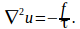## Equation of Surface of Horizontal Membrane Distorted by a Vertical Force at the Origin

A membrane is stretched in the xy plane and pushed upby a force at the origin. Let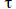bethe surface tension, force per unit length, in the membrane. If a cutis made in the surface, the edges of the cut will tend to move awayfrom each other as a result of this.

Consider a piece of the membrane with length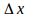andwidth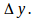Theforces are acting along each edge. The force acting along edge 1is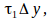atan angle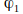tothe xy plane and along edge 2 the force is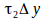atan angle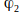to the surface.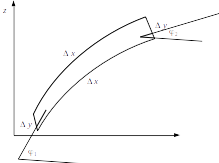The total vertical force along these edges is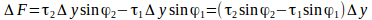If%phi is smaill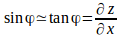sowe can write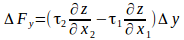Also, for small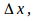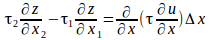Hence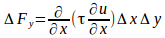The contribution from edges 3 and 4 is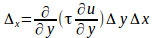Hence the total force is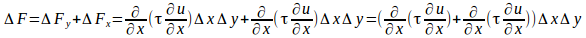If the membrane is in equilibrium then the externalforce per unit area is balanced by this net force per unit area hence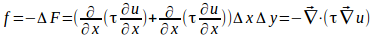Ifisconstant then